Mobile QR Code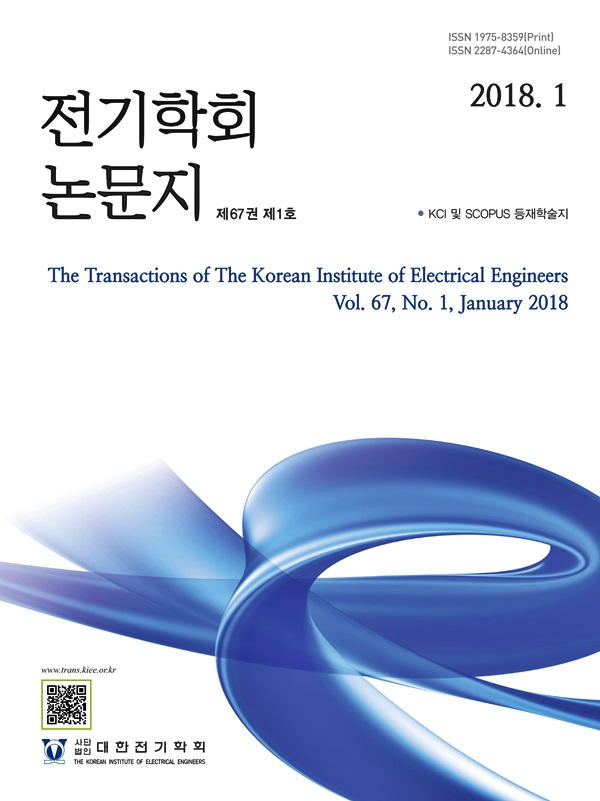The Transactions of the Korean Institute of Electrical Engineers

ISO Journal TitleTrans. Korean. Inst. Elect. Eng.

1. (Dept. of Electrical and Information Engineering, Seoul National University of Science and Technology, Korea)

Mecanum-wheeled omnidirectional mobile robot, path-following, nonlinear model predictive control, ROS

1. Introduction

A mecanum-wheeled omnidirectional mobile robot (MWOMR) is one type of omnidirectional mobile robot which is suitable for application in a narrow environment where a nonholonomic mobile robot movement might be limited. On the other hand, MWOMR can work with that environment because mecanum wheels have a different wheel shape compared to common wheels. They are made up of rollers which are augmented around the wheel with angle $+-45^{\circ}$. These kinds of wheels allow a mobile robot with mecanum wheels to remove steering systems.

A mecanum-wheeled omnidirectional mobile robot is expected to be able to follow varying references with a specified goal. There are two kinds of problems that track varying references: trajectory tracking and path following. Trajectory tracking and path-following problems can track varying references using a different approach. In trajectory tracking, the robot has to follow a time-dependent signal while path-following’s main objective is to track a geometric curve with the timing being a secondary interest (1). The performance difference between trajectory tracking and path-following is presented in (2).

For a mecanum-wheeled omnidirectional mobile robot, several efforts have been taken to control the movement of the robot. In (3)-(4), an approach adapting sliding mode is presented to track a trajectory for MWOMR, which does not consider any constraints that might arise from robot dynamics and environment limitations. (5) has taken an effort in formulating model predictive control for a holonomic mobile robot including MWOMR but focuses only on setpoint stabilization which does not follow varying references.

Implementation of a control method to a real robot has been getting huge attention in the literature. The most important problem arising in real hardware implementation is the robot localization which is crucial in order to define the robot position. (6) shows the results of combining the camera and encoder data to measure the position of the robot and apply an explicit model predictive control to drive the robot to the desired trajectory.

This paper proposes a control framework to minimize the position error of a mecanum-wheeled omnidirectional mobile robot to its desired path in real hardware. A modified nonlinear model predictive path-following control is proposed to ensure that the robot moves in the desired path (7). To implement the NMPC in a real MWOMR system, the combination of lidar, encoder, and IMU is used to obtain the robot position. The tracking performance of NMPC and average execution time per iteration are evaluated to see whether it is applicable to a real robot or not.

The rest of the paper is organized as follows. In section 2, a brief overview of the dynamics of a mecanum-wheeled omnidirectional mobile robot and path-following problem definition are described. In the third section is presented the MPC for path-following of a mecanum-wheeled omnidirectional mobile robot and the implementation in hardware using ROS. In section 4, the proposed NMPC framework is validated in simulation and experiment. In section 5, the results of MPC for path-following performance in the mecanum-wheeled mobile robot are presented. Finally, some conclusions are drawn in the final section.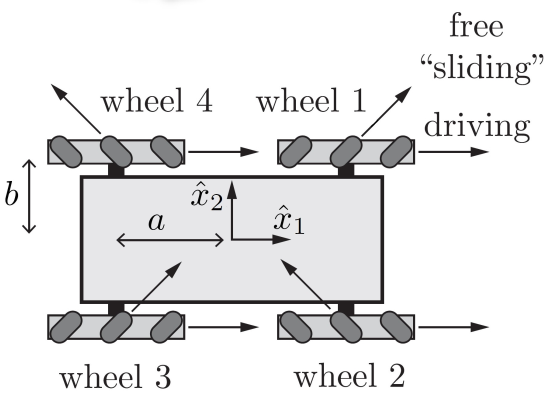2. Problem Definition

In this section, the dynamic model of a mecanum-wheeled omnidirectional mobile robot and the constraint describing the physical limit of the robot speed are introduced. Furthermore, the path-following problem definition and goals are presented.

2.1 Kinematics of MWOMR

The dynamics of MWOMR can be described by

(1)
$\dot x_{t}=f(x_{t},\:u_{t})=R(\theta_{t})· u_{t},\:$

where the states $x=\left[p_{x}, p_{y,}, \theta\right]^{T} \in \mathbb{R}^{n}$ consist of the position of the mobile robot in cartesian coordinate $[p_{x,\:}p_{y}]^{T}$ (m,m) and the orientation angle $\theta$ (rad), and the inputs $u=\left[v_{x}, v_{y}, \omega\right]^{T} \in \mathbb{R}^{m}$ are the linear speed $v_{x}$ (m/s), the lateral speed $v_{y}$ (m/s), and the angular speed $\omega$ (rad/s) of the robot. Rotation matrix $R(\theta_{t})$ in eq(1) is denoted as

(2)
$R(\theta_{t})=\begin{pmatrix}\cos(\theta_{t})&&-\sin(\theta_{t})&&0\\\sin(\theta_{t})&&\cos(\theta_{t})&&0\\0&&0&&1\end{pmatrix}.$

The configuration of all wheels in MWOMR is essential as it determines the movement of MWOMR. Fig 1 illustrates the proper orientation of each wheel. In MWOMR, a motor drives each wheel separately so it can move in any direction freely. The relation of the motor’s angular speed and the input vector $u$ is given by

(3)
$\omega_{m}=\dfrac{1}{r}· Hu ,\:$

where

$H =\begin{pmatrix}1&& -1&& -a-b \\ 1&&1 &&a+b \\ 1&&-1 &&a+b \\ 1&& 1&&-a-b\end{pmatrix}.$ $a$ (m) and $b$ (m) are the half of robot’s wheel base and half of robot’s wheel track, respectively. $w_{m} \in \mathbb{R}^{4}$ (rad/s) is a vector which describes the angular speed of each motor matching the setup in Fig 1 and $r$ (m) is the wheels’ radius. The full derivation of the mecanum-wheeled omnidirectional mobile robot is presented in (8).

From the relation of each motors’ speed and robot’s speed in eq(3), the robot velocity constraint is constructed as introduced in (5),

(4)
$U:=\left\{u \in \mathbb{R}^{3} \mid\|H u\|_{\infty} \leq r \bar{\omega}\right\}$

where

$\|H u\|_{\infty}:=\left|v_{x}\right|+\left|v_{y}\right|+(a+b)|\omega| .$

This paper assumes that the states are bounded by a compact set

(5)
$X=\left\{(p_{x,\:}p_{y,\:}\theta)\in\vec{R}^{3}|x\in[\underline p_{x},\:\bar{p_{x}}],\:y\in[\underline p_{y},\:\bar{p_{y}}],\:\theta\in[-\pi ,\:\pi]\right\}.$

2.2 Path-Following Problem

There are particularly three types of control problems in order to steer the system to the desired point: setpoint stabilization, trajectory tracking, and path-following. The setpoint stabilization is a problem to drive the system states to a constant reference, whereas trajectory tracking and path-following steer the states to follow varying references.

The objective of the trajectory tracking is to follow a certain reference in a specified time. This may limit the accuracy because it does not incorporate the hardware limit that is already stated in eq(4) and eq(5). In contrast, the goal of path-following is to reach and follow a geometric path that does not depend on the time variable. Path-following does not require the system to be at one point in a specific time.

In the path-following, the references to be tracked is denoted as path $P$ which can be defined as a set of points $r$ parameterized by a dimensionless $s$

(6)
$P=\left\{r\in\vec{R}^{n}|s\in[s_{0},\:s_{1}]\mapsto r=p(s)\right\}$

where the $s$ is called a path parameter. The map $p: \mathbb{R} \mapsto \mathbb{R}^{n}$ is assumed to be continuously differentiable. Note that the time evolution of $s(t)\in[s_{0},\:s_{1}]$ is not known a priori, but it is another degree of freedom to choose in this problem. Here, a virtual input $\phi_{t}$ is chosen to parameterize the evolution of $s_{t}$ as

(7)
$\dot s_{t}=\phi_{t}.$

In the path-following, the controller is designed to achieve these conditions (1):

i) Path convergence

$\lim _{t \rightarrow \infty}\left\|x_{t}-p\left(s_{t}\right)\right\|=0$

ii) Forward motion

$\dot{s}_{t} \geq 0$ and $\lim _{t \rightarrow \infty}\left\|s_{t}-s_{1}\right\|=0$

iii) Constraint satisfaction

$x_{t} \in X, u_{t} \in U \quad \forall t \in\left[t_{0}, \infty\right)$

Condition i) ensures that the system moves along the desired path. Condition ii) makes sure that the robot is moving forward along the path. The last condition guarantees that the system follows the hardware limitations.

3. Model Predictive Path-Following Control

In this paper, a discrete-time system of MWOMR dynamics eq(1) and path parameter evolution eq(7) are considered to be used in the model predictive control. A simple Euler’s formula is used to discretize the system and path parameter evolution as

(8a)
$x_{k+1}=x_{k}+t_{s}· f(x_{k},\:u_{k}),\:$

(8b)
$s_{k+1}=s_{k}+t_{s}·\phi_{k}$

where $t_{s}$ is the sampling time.

3.1 MPC Formulation for Path-Following

In order to calculate the optimal input for path-following problem, the following cost function is considered

(9)
\begin{aligned} J\left(x_{k}, s_{k}\right)=\sum_{j=0}^{N-1}(&\left\|x_{k+j \mid k}-p\left(s_{k+j \mid k}\right)\right\|_{Q}^{2}+\left\|u_{k+j \mid k}\right\|_{R}^{2} \\ &\left.+\left\|s_{k+j \mid k}-s_{1}\right\|_{P}^{2}\right), \end{aligned}

where $P,\: Q,\:$ and $R$ are positive definite and $(·)_{k+j | k}$ means the predicted value at time step $k+j$ based on the value measured at time step $k$. The first term of eq(9) ensures that the system tracks the specified path, and the third term forces the robot to move forward along the specified path.

Suppose that $bold{u}=\left\{u_{k|k},\: u_{k+1|k},\:\ldots ,\: u_{k+N-1|k}\right\}$ and $bold\Phi =\left\{\phi_{k|k},\:\phi_{k+1|k,\:}\ldots ,\:\phi_{k+N-1|k}\right\}$ denote the sequences of all predicted inputs and virtual inputs, respectively. Assume that $x_{0}=\left[p_{x},\: p_{y},\:\theta\right]^{T}$ and $s_{0}$ are given, the MPC control law is obtained by solving the following optimization problem

(10a)
\begin{aligned}\min \\ bold{u},\:\Phi\end{aligned}J(x_{k,\:}s_{k})

subject to

(10b)
$x_{k+j+1|k}=x_{k+j|k}+t_{s}· f(x_{k+j|k},\:u_{k+j|k}),\:$ $x_{k|k}=x_{0}$,

(10c)
$s_{k+j+1|k}=s_{k+j|k}+t_{s}·\phi_{k+j|k}$, $s_{k|k}=s_{0}$,

(10d)
$\phi_{k+j|k}\ge 0$,

(10e)
$x_{k+j+1|k}\in X,\: u_{k+j|k}\in U$,

(10f)
$s_{k+j+1|k}\in[s_{0,\:}s_{1}]$.

The first element of sequence $bold{u}$, $u_{k|k}$, is applied to the robot and $s_{k+1|k}$ becomes the next initial value $s_{0}$ of problem eq(10).

In the case where no initial condition for parameter $s$ is given when $t=0$, $s_{t_{0}}$ can be obtained by solving the following optimization problem:

(11)
$s_{t_{0}}=\underset{s}{\operatorname{argmin}} \in\left[s_{0}, s_{1}\right]\left\|x_{0}-p\left(s_{t_{0}}\right)\right\|$

3.2 MPC Implementation in ROS

Since MPC needs the state of the robot to solve the optimization problem, knowing the robot position and orientation angle becomes a critical issue. To measure the state of the robot, this work uses three kinds of sensor to get the robot position and rotation angle: encoder, IMU, and lidar. The data from each sensor is fused using EKF localization inside a robot localization package in ROS (9). The results of the localization are the robot position and orientation angle and can be accessed through ROS localization nodes.

After collecting the required information for the optimization problem, the MPC problem can be solved directly from the robot. In ROS, the MPC problem is defined using CasADi as a tool to model nonlinear optimizations (10). CasADi creates an optimization object only once before the robot starts. Then, the optimal control input can be computed by applying the IPOPT algorithm to solve the optimization problem eq(10) (11).

The optimal control input, which is the robot speed commands, is passed through the robot and converted to wheels’ angular speed by eq(3). The MPC implementation in MWOMR using ROS is illustrated in Fig 2.

4. Simulation and Experiment Setup

In this section, the setup for validating the MPC formulation in simulation and hardware experiment is presented. Two kinds of paths are used to examine the MPC performance for the path- following problem in MWOMR. Both simulation and hardware experiment deal with circle path and 8-shaped path as described in eq(12) and eq(13), respectively,

(12)
$p(s_{t})=\begin{bmatrix}p_{x,\:path}\\p_{y,\:path}\\\theta_{path}\end{bmatrix}=\begin{bmatrix}\cos(2\pi s_{t})-1\\\sin(2\pi s_{t})\\{a}\tan 2(p_{x_{e}},\:p_{y_{e}})\end{bmatrix},\:$

(13)
$p(s_{t})=\begin{bmatrix}p_{x,\:path}\\p_{y,\:path}\\\theta_{path}\end{bmatrix}=\begin{bmatrix}1.8\sin(2\pi s_{t})\\1.2\sin(2· 2\pi s_{t})\\{a}\tan 2(p_{x_{e}},\:p_{y_{e}})\end{bmatrix},\:$

where $p_{x_{e}}$ and $p_{y_{e}}$ are the differences between the reference and current position and $s\in[0 ,\:1]$.

Since MWOMR can move in any direction without changing the orientation angle, the performance comparison between the robot moving with fixed and varying orientation angle is evaluated. Varying the orientation angle is considered in the path formulation eq(12) and eq(13). In order to reach a path with a fixed orientation angle, $\theta_{path}$ can be assigned to a constant $c$ (rad) as the desired angle of the robot.

Fig. 3. (a) Mobile robot model in Gazebo, (b) Experimental mobile robot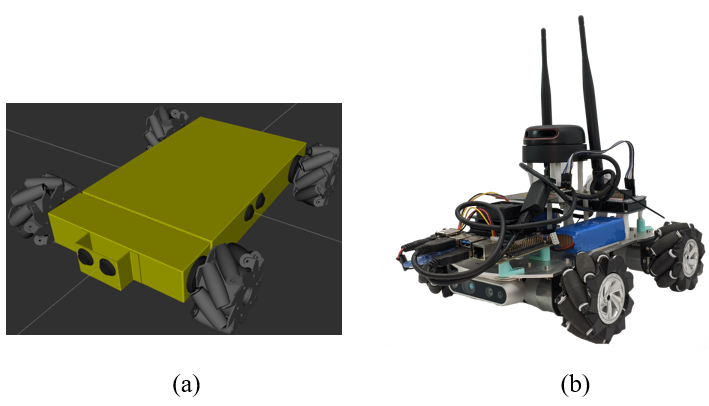4.1 Simulation Setup

The simulation is performed in the GAZEBO simulator using Nexus Robot 4WD mecanum wheel mobile robot model as illustrated in Fig 3a. The simulation is carried out in Ubuntu 18.04 LTS in a computer with AMD Ryzen 3950X. Table 1 summarizes all parameters and weights used in NMPC formulation for simulation.

Table 1. Robot parameters and weights for simulation

 Parameters Value Wheel track 0.3 m Wheel base 0.3 m Wheel's radius 0.05 m Maximum wheel's speed 12 rad/s Q weights diag([20,20,10]) R weights diag([5,5,5]) P weights 15 MPC prediction horizon 15

4.2 Experiment Setup

Jetson Nano 4GB is installed on the robot to solve the MPC optimization problem. The picture of the developed MWOMR is given in Fig 3b. The robot’s parameters and weights are given in Table 2.

Table 2. Robot parameters and weights for experiment

 Parameters Value Wheel track 0.215 m Wheel base 0.165 m Wheel's radius 0.039 m Maximum wheel's speed 33 rad/s Q weights diag([15,15,10]) R weights diag([5,5,5]) P weights 10 MPC prediction horizon 15

5. Results

In this section, the performance of the proposed method with a circle and 8-shaped path is presented. First, the proposed method is validated if it can follow a circle and 8-shaped trajectories in simulation. The results of tracking performance of both paths are shown in Fig 4 and Fig 5, respectively. From the figures, it is verified that MPC path-following can steer the robot to move along the specified path while satisfying the robot dynamics and constraints.

In order to look into the robustness of the proposed design against load variations, the NMPC based tracking controller is applied to the circle shape reference trajectory with three different loads: no load, 20 kg load, and 40 kg load. Fig 6 demonstrates that the proposed NMPC results in acceptable tracking performance with different loads.

Next, the experiment results are presented when the method is applied to the real hardware using two kinds of paths with fixed and varying orientation angle. The results of a circle and 8-shaped paths are shown in Fig 7 and Fig 8, respectively. From the figures, the robot could follow the path sufficiently well with both fixed and varying orientation angle.

In addition to tracking performance, the average time execution per iteration is given in order to show that the computation time, both in simulation and real hardware, is less than the robot sampling time. The sampling time is set to 0.1 s which adopts the sampling time in the real robot lidar. The average execution time taken for completing one iteration is summarized in Table 3.

Table 3. Average execution time

 Case Average time in simulation (Ryzen 3950X) Average time in real robot (Jetson Nano) Circle path with fixed orientation 3.476 ms 31.579 ms Circle path with varying orientation 3.010 ms 36.184 ms 8-shaped path with fixed orientation 3.153 ms 31.074 ms 8-shaped path with varying orientation 3.355 ms 36.072 ms

Fig. 7. (a) Tracking circle path with fixed orientation; (b) Tracking circle path with varying orientation. Arrows indicate the orientation angle of the robot.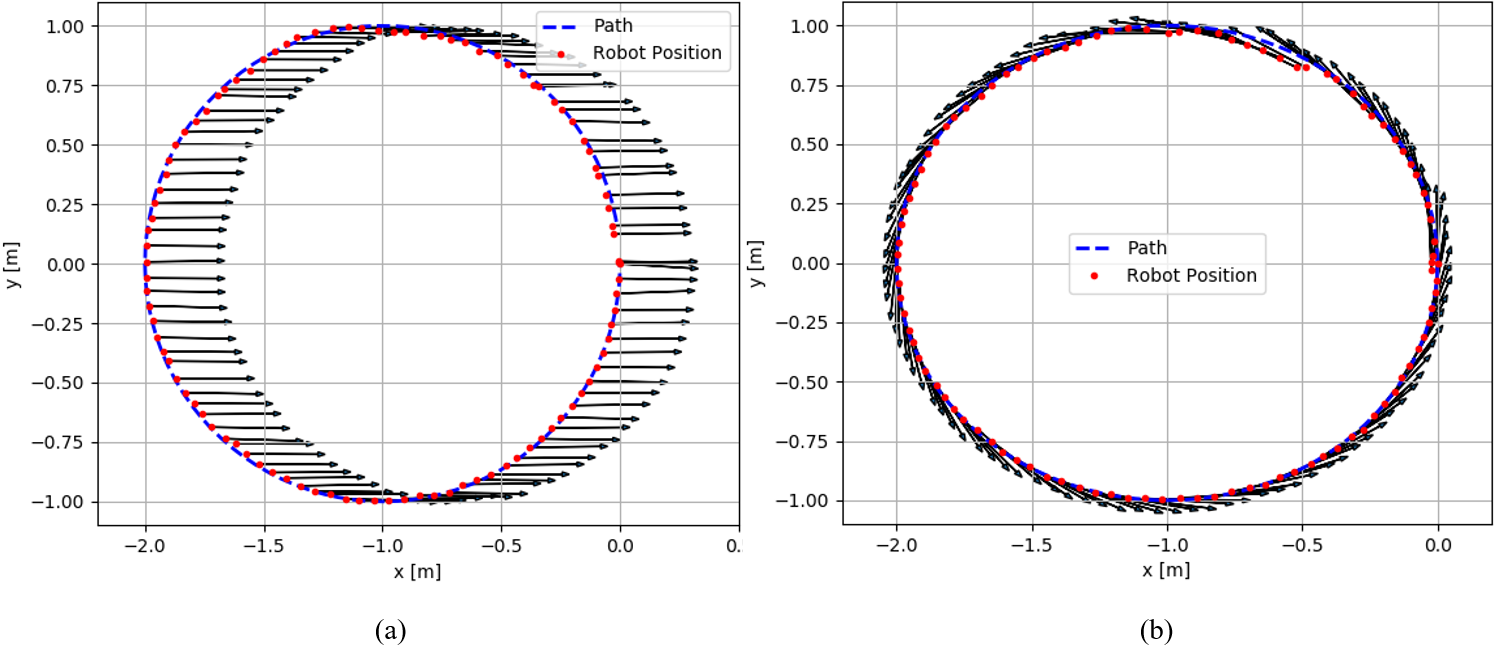Fig. 8. (a) Tracking 8-shaped path with fixed orientation; (b) Tracking 8-shaped path with varying orientation. Arrows indicate the orientation angle of the robot.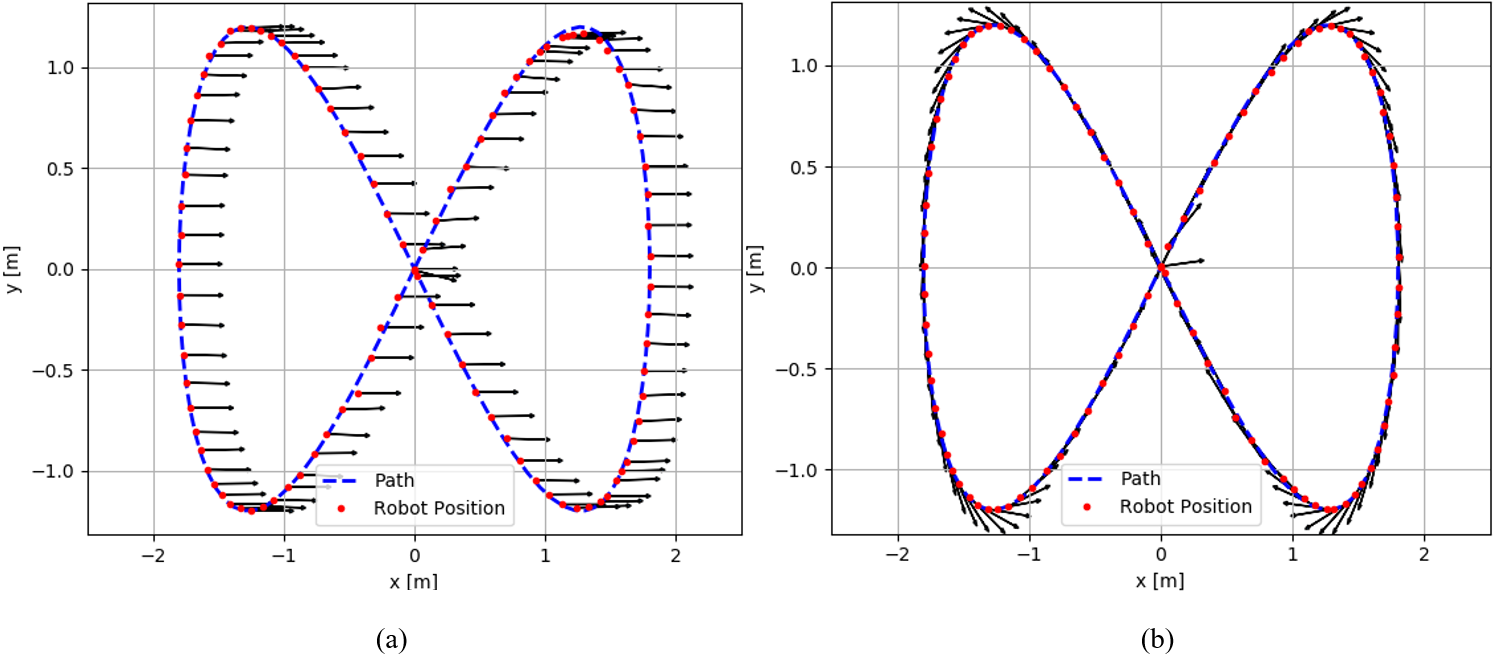6. 결 론

In this paper, a control framework for mecanum-wheeled mobile robot using model predictive control is presented. To this end, a nonlinear model predictive path-following control is designed for the mobile robot first. Then, real hardware setup is developed in which the designed MPC is implementable with several sensors. Simulation and experiment results show that the mobile robot tracks given trajectories successfully while satisfying all the physical constraints. Moreover, the optimization problem for MPC can be solved during two consecutive system sampling times in real embedded systems.

Future work along this direction includes considering a speed reference as well in the middle of path following of the mecanum-wheeled mobile robot.

Acknowledgements

This work was supported by the Industrial Fundamental Technology Development Program (No. 20014786, Development of AI-based Concrete Slab Finishing Automation System) funded by the Ministry of Trade, Industry & Energy (MOTIE) of Korea

References

1
J. Matschek, T. Bäthge, T. Faulwasser, R. Findeisen, 2019, Nonlinear Predictive Control for Trajectory Tracking and Path Following: An Introduction and Perspective, in Handbook of Model Predictive Control S. V Raković and W. S. Levine Eds. Cham: Springer International Publishing, pp. 169-1982
A. P. Aguiar, J. P. Hespanha, P. V Kokotovic, 2005, Path- following for nonminimum phase systems removes performance limitations, IEEE Trans. Automat. Contr., Vol. 50, No. 2, pp. 234-2393
Z. Sun, S. Hu, D. He, W. Zhu, H. Xie, J. Zheng, 2021, Trajectory-tracking control of Mecanum-wheeled omnidirectional mobile robots using adaptive integral terminal sliding mode, Comput. Electr. Eng., Vol. 96, pp. 1075004
Z. Sun, H. Xie, J. Zheng, Z. Man, D. He, 2021, Path- following control of Mecanum-wheels omnidirectional mobile robots using nonsingular terminal sliding mode, Mech. Syst. Signal Process., Vol. 147, pp. 1071285
M. W. Mehrez, K. Worthmann, J. P. V Cenerini, M. Osman, W. W. Melek, S. Jeon, 2020, Model Predictive Control without terminal constraints or costs for holonomic mobile robots, Rob. Auton. Syst., Vol. 1276
Y. Jin, S. Han, S. Lee, May 2019, Implementation of tracking control system for autonomous mobile robot based on state estimation with multi-sensor, Trans. KIEE, Vol. 68, No. 5, pp. 678-6847
T. Faulwasser, R. Findeisen, 2016, Nonlinear Model Predictive Control for Constrained Output Path Following, IEEE Trans. Automat. Contr., Vol. 61, No. 4, pp. 1026-10398
K. M. Lynch, F. C. Park, 2017, Modern Robotics, Cambridge University Press9
T. Moore, D. Stouch, 2016, A Generalized Extended Kalman Filter Implementation for the Robot Operating System, in Intelligent Autonomous Systems 13, pp. 335-34810
J. A. E. Andersson, J. Gillis, G. Horn, J. B. Rawlings, M. Diehl, 2019, CasADi: a software framework for nonlinear optimization and optimal control, Math. Program. Comput., Vol. 11, No. 1, pp. 1-3611
A. Wächter, L. T. Biegler, 2006, On the implementation of an interior-point filter line-search algorithm for large-scale nonlinear programming, Math. Program., Vol. 106, No. 1, pp. 25-57저자소개

He received his B.E. degree in Electrical Engineering from Universitas Indonesia, Depok, Indonesia in 2018.

He is a Ph.D. candidate at the Department of Electrical and Information Engineering, Seoul National University of Science and Technology (SeoulTech).

His research interests include optimal control, learning-based control, and its application to robotics.

He received B.S,, M.S. and Ph.D. degrees in electrical engineering from Korea University, Seoul, South Korea, in 1998, 2000, and 2005, respectively.

He was a postdoctoral researcher at Seoul National University in South Korea, the University of Stuttgart in Germany and the University of Leicester in the UK from March 2005 to February 2006, from March 2006 to December 2007, from January 2008 to January 2009 respectively.

He is currently a full professor in the Dept. of Electrical and Engineering Information at Seoul National University of Science and Technology, Seoul, South Korea since 2009.

His research interests include model predictive control, distributed control, application of deep learning to energy systems and robotics.